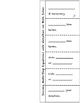# Multi-Step Equations with One Solution FoldablesSubject
Resource Type
File Type
PDF (1 MB|8 pages)
Standards
\$1.50
• Product Description
• Standards
Use these two foldables to help your students understand the steps involved in solving a multi-step equation with one solution. One foldable can be used for multi-step equations with variables on one side. The other can be used for multi-step equations on both sides. Feel free to use your own examples, or use the examples included.
Solve linear equations with rational number coefficients, including equations whose solutions require expanding expressions using the distributive property and collecting like terms.
Give examples of linear equations in one variable with one solution, infinitely many solutions, or no solutions. Show which of these possibilities is the case by successively transforming the given equation into simpler forms, until an equivalent equation of the form 𝘹 = 𝘢, 𝘢 = 𝘢, or 𝘢 = 𝘣 results (where 𝘢 and 𝘣 are different numbers).
Total Pages
8 pages